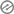# Revision MATHEMATICS BASIC SIX SECOND TERM

FIRST TERM EXAMINATION 2019/2020

CLASS: PRIMARY 6                                                                     SUBJECT: MATHEMATICS

NAME:……………………………………………………………………………………

Answer all the questions1. Write in figures: One million, one thousand and eleven

(a) 1,010,011 (b) 1100111 (c) 1,001,011 (d) 10101011

1. Write CLXII in Hindu Arabic figures (a) 116 (b) 162           (c) 142           (d) 199
2. What is the value of (1.7)2 (a) 28.9    (b) 2.89     (c) 0.289      (d) 289

4.126 x .04  (a) 0.54      (b) 0.50      (c) 0.00504       (d) 0.1305.Subtract 0.07 from 1.1 (a) 1.3     (b) 1.03      (c) 0.13       (d) 1.3

6.If V + 18 = 62, what is the value of V (a) 44     (b) 62     (c) 54       (d) 807.Express 50 as a decimal of 200  (a) 0.25      (b) 0.005     (c) 0.05       (d) 0.51

8.Decrease N1000 by 10% (a) N900     (b) N10      (c) N110      (d) N800

9.Calculate the simple interest on N500 for 5years at the rate of 5% per annum

(a) N50     (b) N500       (c) N25          (d) N125

10.What fraction of 2 minutes is 6 seconds (a) 1/6 (b) 1/10     (c) 1/20     (d) 2/611.Which of the following is not a prime number (a) 2  (b) 3     (c) 4     (d) 5

12.Write out all factors of 8 (a) 1, 2, 3, 4    (b) 1, 2, 4, 8     (c) 8, 16, 24, 32      (d) 2, 4, 6, 8

1. In a class of 50 pupils, 70% passed in the final exams. What percentage failed _________ %

(a) 120      (b) 20      (c) 30     (d) 100

13.What is 25% of 100 (a) 25     (b) 50     (c) 100     (d) 12514.Divide 8.107 by 6.05 (a) 0.344     (b) 0.442    (c) 1.34     (d) 1.571

15.How many prime numbers are there from 1 to 100 (a) 16 (b) 100     (c) 26     (d) 50

16.Express 70 kobo as a decimal of N1 (a) 0.75  (b) 0.70     (c) 0.35     (d) 0.1

17.If 3 books cost N225, what is the cost of 5 similar books (a) N375 (b) N350 (c) N300 (d) 13518.From N05 take away N30.99 (a) N5.16 (b) N5.09 (c) N4.09 (d) N4.16

19.What is 40% of N00 (a) N20.00 (b) N200.00 (c) N2.00 (d) 20k

Section B: Show all Workings1.Convert from base ten to base two (a) 77ten (b) 100ten

2.Write out the following figures as products of their prime factors (a) 160 (b) 288

3.Write out all factors of these figures (a) 73 (b) 274.Find the sum of these figures in base two

(a)   1 0 1 two                                            (b) 1 0 1 0 two

•         1 1 two                                             +       1 1 0 two5.Calculate the H.C.F of the following figures (a) 24 and 48 (b) 50 and 60

SECOND TERM EXAMINATION 2021 MATHEMATICS PRIMARY 6Second Term Examination 2020 Academic Year Primary Six Basic 6 Mathematics

SECOND TERM EXAMINATION  PRIMARY 6 MATHEMATICSSECOND TERM EXAMINATION 2019 CLASS: PRIMARY 6 SUBJECT: MATHEMATICS NAME:……………………………………………………………………………………